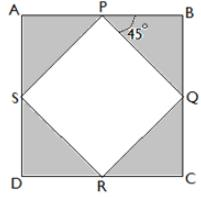# Test: Problem Solving- 2

## 10 Questions MCQ Test GMAT Mock Test for Practice | Test: Problem Solving- 2

Description
This mock test of Test: Problem Solving- 2 for GMAT helps you for every GMAT entrance exam. This contains 10 Multiple Choice Questions for GMAT Test: Problem Solving- 2 (mcq) to study with solutions a complete question bank. The solved questions answers in this Test: Problem Solving- 2 quiz give you a good mix of easy questions and tough questions. GMAT students definitely take this Test: Problem Solving- 2 exercise for a better result in the exam. You can find other Test: Problem Solving- 2 extra questions, long questions & short questions for GMAT on EduRev as well by searching above.
QUESTION: 1

### A square PQRS is enclosed in another square ABCD. Find the ratio of the area of PQRS to the area of ABCD.Solution:

If we divide the figure in 4 equal parts we see that the shaded area is half the total area.

An alternate approach is to find the unshaded area by observing that it is composed of right angled triangles, adding this area and subtracting from total can get the shaded area.

QUESTION: 2

### What is the ratio of the area of triangle ABC to the area of square ADFC if CB=(CF)/4Solution:

We find the area using the one fourth length, 0.5*base*height, then divide it by the area of the square (square of side). For length we use an arbitrary variable, but the choice doesn't matter as the ratio is independent of it.

Let's suppose that the length of one side is 'x'. Then area of square becomes x2. The area of triangle becomes (1/2)(x)( x/4) = x2/8. So, the ratio becomes (x2/8)/x2 which simplifies to 1/8

QUESTION: 3

### If the product of two integers x and y is less than 82 with y being a multiple of three. What is the highest value that x may have?

Solution:

In order to find the maximum value of x, we need to put the minimum value of y i.e. 3. Now, if we divide 82 by 3, we get 27.33, which means the maximum integer value that x can have must be less than 27.33. From the given options, we choose option C which is 27.

QUESTION: 4

Adam is 2 years older than Mike. The square of Adam’s age is 28 greater than the square of Mike’s age in years. What is the sum of Adam’s age and Mike’s age?

Solution:

Let suppose that Adam's age is 'A', and Mike's age is 'M'. From the statement of the questions, we deduce following two equations.

A = 2+M and also A2= M2+28 -> A2 - M2 = 28 -> (A+M)(A-M)=28 and then putting

A-M=2, we get (A+M) = 14. Therefore, the sum of Adam's age and Mike's age is 14.

QUESTION: 5

Adam has bought a certain number of apples. Jen has bought 5 times the fruit that Adam has bought. If Jen has bought two and a half dozen apples how many apples does Adam have?

Solution:

It's a relatively simple question. Jen has five times the number of apples which Adam has. If Jen has 30 apples, Adam has 30/5=6 apples.

QUESTION: 6

What would be the circumference of a circle that has been inscribed in a square of area 5.

Solution:

Area of a square is multiple of any two sides. If the area if 5, each side of the square has to be √5 i.e. √5*√5=5

Now, the radius of the circle would be half of √5 because the circle in inscribed inside the square. So Radius = √5/2

So, Circumference= 2*( π)*√5/2

Circumference= π√5

QUESTION: 7

What could be the possible value of 'y' after the intersection of points

y= -x2 + 3 and y= x2- 5

Solution:

We equate the two equations, find the two points of intersection of the two functions and compute the distance using the distance between points formula.

After equating these two equations, we get x= +2 and x=-2 which gives us y=7, and y=-1. Therefore, we select option E is as our correct answer.

QUESTION: 8

A house is built by 20 workers in 30 days. How many workers will be needed to complete the work in 15 days?

Solution:

As stated in the question, the work needs to be completed in half the days as compared to the earlier timeline for completion, therefore, the number the workers needs to be doubled.

QUESTION: 9

Master Chef Alan makes a dish every day from one of his recipe books. He has written 3 books and each book contains 15 different recipes. What is the probability that he will cook 4th dish from 3rd book today?

Solution:

We note that there are three books and each book contains 15 recipes so there are a total of 45 recipes. The probability that he will cook 4th dish from 3rd book today is 1/45. It's important that you must not get distracted by irrelevant information. All the recipes are different from each other.

QUESTION: 10

In a Christmas sale, the prices of Dell Laptops were reduced by 10% for public. However, for Dell employees, the price was further reduced by 5%. If the original price of a laptop was \$330 before Christmas sale, approximately how much would it cost in a Christmas sale to a Dell employee?

Solution:

Original Price = \$330

Price after 10% discount= 330 * 0.9 = \$297

Price after further 5% discount (for Dell employees) = 297* 0.95 = \$282. Therefore, Option C.